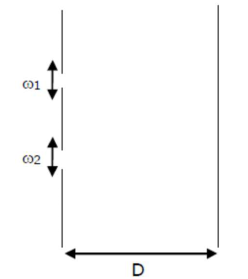# In a Young's double slit experiment,`
Question:

In a Young's double slit experiment, the width of the one of the slit is three times the other slit. The amplitude of the light coming from a slit is proportional to the slit-width. Find the ratio of the maximum to the minimum intensity in the

1. $4: 1$

2. $2: 1$

3. $3: 1$

4. $1: 4$

Correct Option: 1

Solution:

(1)given $: \omega_{2}=3 \omega_{1}$

also, $A \propto \omega$

$\frac{\omega_{1}}{\omega_{2}}=\frac{1}{3} \cdots(1)$

Assume $\omega_{1}=x, \omega_{2}=3 x$

we know that

$I_{\max }=\left(A_{1}+A_{2}\right)^{2}$, and

$I_{\min }=\left(A_{1}-A_{2}\right)^{2}$

$\frac{A_{1}}{A_{2}}=\frac{\omega_{1}}{\omega_{2}} \ldots(2)$

from equation (2) we can say that

$A_{1}=A$ and $A_{2}=3 A$

Now, $\frac{I_{\max }}{I_{\min }}=\frac{(A+3 A)^{2}}{(A-3 A)^{2}}=\frac{16 A^{2}}{4 A^{2}}=\frac{4}{1}$

$\Rightarrow \frac{I_{\max }}{I_{\min }}=\frac{4}{1}$